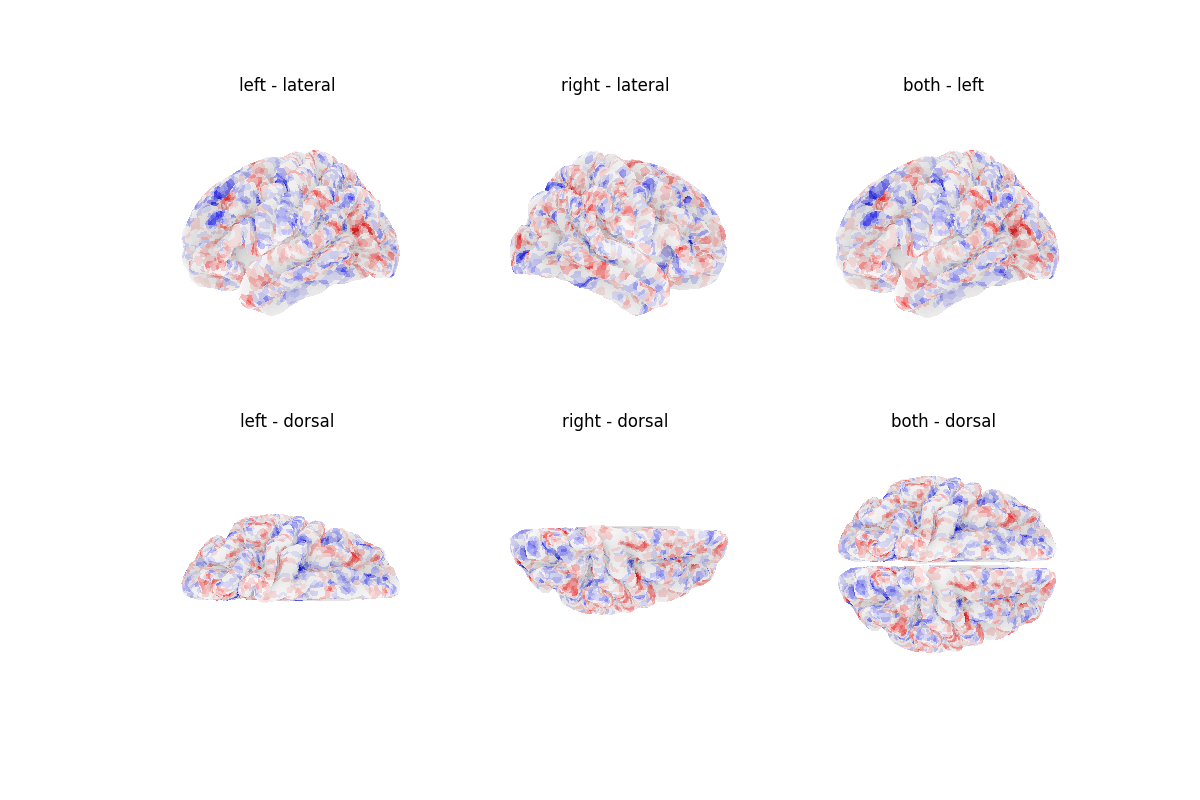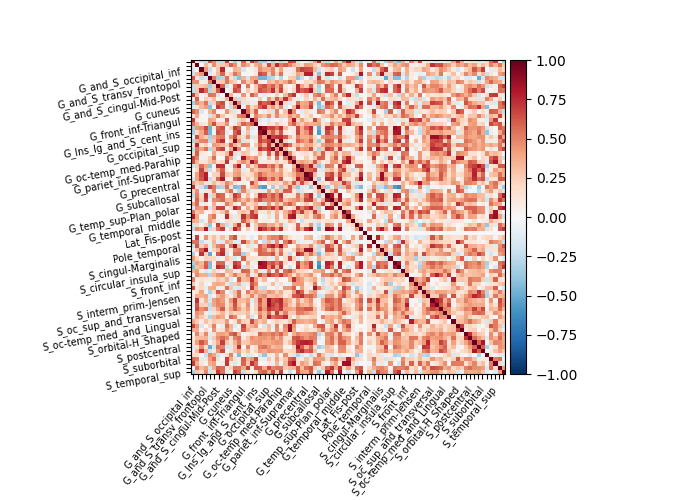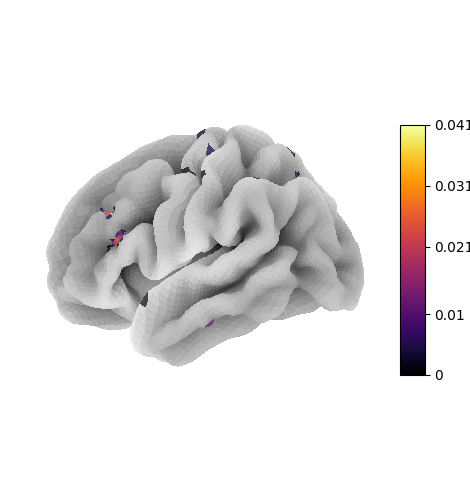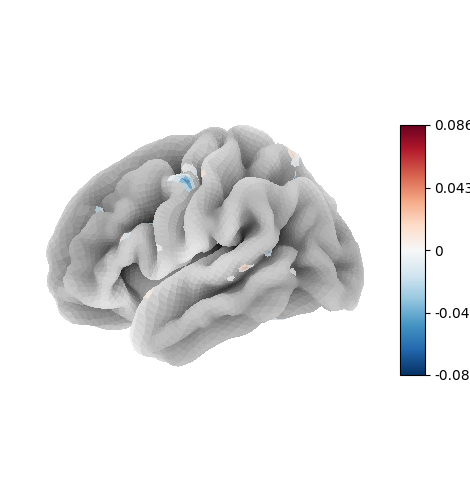# A short demo of the surface images & maskers#

copied from the nilearn sandbox discussion, to be transformed into tests & examples

Note

this example is meant to support discussion around a tentative API for surface images in nilearn. This functionality is provided by the nilearn.experimental.surface module; it is still incomplete and subject to change without a deprecation cycle. Please participate in the discussion on GitHub!

```from typing import Optional, Sequence

from matplotlib import pyplot as plt

from nilearn import plotting
from nilearn.experimental import surface

def plot_surf_img(
img: surface.SurfaceImage,
parts: Optional[Sequence[str]] = None,
mesh: Optional[surface.PolyMesh] = None,
**kwargs,
) -> plt.Figure:
if mesh is None:
mesh = img.mesh
if parts is None:
parts = list(img.data.keys())
fig, axes = plt.subplots(
1,
len(parts),
subplot_kw={"projection": "3d"},
figsize=(4 * len(parts), 4),
)
for ax, mesh_part in zip(axes, parts):
plotting.plot_surf(
mesh[mesh_part],
img.data[mesh_part],
axes=ax,
title=mesh_part,
**kwargs,
)
assert isinstance(fig, plt.Figure)
return fig

img = surface.fetch_nki()
print(f"NKI image: {img}")

print(f"Image mean: {mean_img}")

plot_surf_img(mean_img)
plotting.show()
``````/opt/hostedtoolcache/Python/3.12.0/x64/lib/python3.12/site-packages/nilearn/experimental/__init__.py:10: UserWarning:

All features in the nilearn.experimental module are experimental and subject to change. They are included in the nilearn package to gather early feedback from users about prototypes of new features. Changes may break backwards compatibility without prior notice or a deprecation cycle. Moreover, some features may be incomplete or may have been tested less thoroughly than the rest of the library.

NKI image: <SurfaceImage (895, 20484)>
Image mean: <SurfaceImage (20484,)>
```

## Connectivity with a surface atlas and SurfaceLabelsMasker#

```from nilearn import connectome, plotting

img = surface.fetch_nki()
print(f"NKI image: {img}")

labels_img, label_names = surface.fetch_destrieux()
print(f"Destrieux image: {labels_img}")
plot_surf_img(labels_img, cmap="gist_ncar", avg_method="median")

connectome = (
)

plotting.show()
```
••```NKI image: <SurfaceImage (895, 20484)>
Destrieux image: <SurfaceImage (20484,)>
```

## Using the Decoder#

```import numpy as np

from nilearn import decoding, plotting
from nilearn._utils import param_validation
```

The following is just disabling a couple of checks performed by the decoder that would force us to use a NiftiMasker.

```def monkeypatch_masker_checks():
return screening_percentile

```

Now using the appropriate masker we can use a Decoder on surface data just as we do for volume images.

```img = surface.fetch_nki()
y = np.random.RandomState(0).choice([0, 1], replace=True, size=img.shape)

decoder = decoding.Decoder(
param_grid={"C": [0.01, 0.1]},
cv=3,
screening_percentile=1,
)
decoder.fit(img, y)
print("CV scores:", decoder.cv_scores_)

plot_surf_img(decoder.coef_img_, threshold=1e-6)
plotting.show()
``````/opt/hostedtoolcache/Python/3.12.0/x64/lib/python3.12/site-packages/sklearn/feature_selection/_univariate_selection.py:112: UserWarning:

Features [    8    36    38 ... 20206 20207 20208] are constant.

/opt/hostedtoolcache/Python/3.12.0/x64/lib/python3.12/site-packages/sklearn/feature_selection/_univariate_selection.py:113: RuntimeWarning:

invalid value encountered in divide

/opt/hostedtoolcache/Python/3.12.0/x64/lib/python3.12/site-packages/sklearn/feature_selection/_univariate_selection.py:112: UserWarning:

Features [    8    36    38 ... 20206 20207 20208] are constant.

/opt/hostedtoolcache/Python/3.12.0/x64/lib/python3.12/site-packages/sklearn/feature_selection/_univariate_selection.py:113: RuntimeWarning:

invalid value encountered in divide

/opt/hostedtoolcache/Python/3.12.0/x64/lib/python3.12/site-packages/sklearn/feature_selection/_univariate_selection.py:112: UserWarning:

Features [    8    36    38 ... 20206 20207 20208] are constant.

/opt/hostedtoolcache/Python/3.12.0/x64/lib/python3.12/site-packages/sklearn/feature_selection/_univariate_selection.py:113: RuntimeWarning:

invalid value encountered in divide

CV scores: {0: [0.4991939095387371, 0.5115891053391053, 0.4847132034632034], 1: [0.4991939095387371, 0.5115891053391053, 0.4847132034632034]}
```

## Decoding with a scikit-learn Pipeline#

```import numpy as np
from sklearn import feature_selection, linear_model, pipeline, preprocessing

from nilearn import plotting

img = surface.fetch_nki()
y = np.random.RandomState(0).normal(size=img.shape)

decoder = pipeline.make_pipeline(
preprocessing.StandardScaler(),
feature_selection.SelectKBest(
score_func=feature_selection.f_regression, k=500
),
linear_model.Ridge(),
)
decoder.fit(img, y)

coef_img = decoder[:-1].inverse_transform(np.atleast_2d(decoder[-1].coef_))

vmax = max([np.absolute(dp).max() for dp in coef_img.data.values()])
plot_surf_img(
coef_img,
cmap="cold_hot",
vmin=-vmax,
vmax=vmax,
threshold=1e-6,
)
plotting.show()
```Total running time of the script: (0 minutes 21.641 seconds)

Estimated memory usage: 1530 MB

Gallery generated by Sphinx-Gallery